# Thread: Integration By Parts - Proof

1. ## Integration By Parts - Proof

Hi Guys,

I've had a search for "Integration by parts" and couldn't quite find what I was looking for... so apologies if this is actually somewhere in the forum and I've just missed it.

My Question has two parts.

Firstly a (possibly) silly sanity check...
$\displaystyle \int dx/dy = y + C$
This is correct right?

Assuming this is correct, from the product rule we have

$\displaystyle dy/dy = v.du/dx + u.dv/dx$

So if we integrate both sides we have

LHS = y = uv

But I'm puzzled by the integration of the RHS

The proof I'm reading gives

$\displaystyle RHS = \int u.dv/dx.dx + \int v.du/dx.dx = \int u.dv + \int v.du$
So, where I'm a little stuck is why
$\displaystyle \int u.dv/dx.dx \neq uv$
I.e why does
$\displaystyle \int dv/dx.dx \neq v?$

Any help would be much appreciated. Sorry for the rubbish latex btw, most of the little image things under the latex cheat sheet haven't loaded.

James

2.Originally Posted by jameshume$\displaystyle \int dx/dy = y + C$
This is correct right?
Not quite sure what the even means.

Think on the Product Rule of Differentiation.

Given u(x) and v(x), we have d(u*v) = u*dv + v*du.

Simply rearrange. d(u*v) - u*dv = v*du

Antiderivatives with respect to x

$\displaystyle \int d(u*v) - \int u*dv = \int v*du$

or

$\displaystyle u*v - \int u*dv = \int v*du$

which should look familiar.

3.Originally Posted by TKHunnyNot quite sure what the even means.

Think on the Product Rule of Differentiation.

Given u(x) and v(x), we have d(u*v) = u*dv + v*du.

Simply rearrange. d(u*v) - u*dv = v*du

Antiderivatives with respect to x

$\displaystyle \int d(u*v) - \int u*dv = \int v*du$

or

$\displaystyle u*v - \int u*dv = \int v*du$

which should look familiar.
Hi TKH,

Thanks for your reply. Couple of typos there I hadn't spotted in my original post so no wonder your confusion at the first satement...
$\displaystyle \int \frac{dy}{dx} dx = y + C$

Starting with the product rule

$\displaystyle \frac{d}{dx}(uv) = v\frac{du}{dx} + u\frac{dv}{dx}$

So, again I made a mistake in what I had written, kinda, in that I should have written it as above, where y = uv.

So I would expect
$\displaystyle \int \frac{d}{dx}(uv) dx = uv$

I'm happy with that bit...

What I don't really get is
$\displaystyle \int u\frac{dv}{dx} dx$ becomes $\displaystyle \int u dv$?

I had a slight realisation when writing this and realised why it couldn't become $\displaystyle uv$, but equally I don't understand the apparent cancelling of the "d" terms. I could understand this if they were $\displaystyle \delta$s and therefore could be algebraically manipulated in this way.

I'm gonna go back to have a little think, but if you have any illuminating answers in the mean time I'd love to hear them.

THanks agin,
James

4. You can always do without the fractional notation, which can be misleading anyway (but useful, too!). Just in case a picture helps...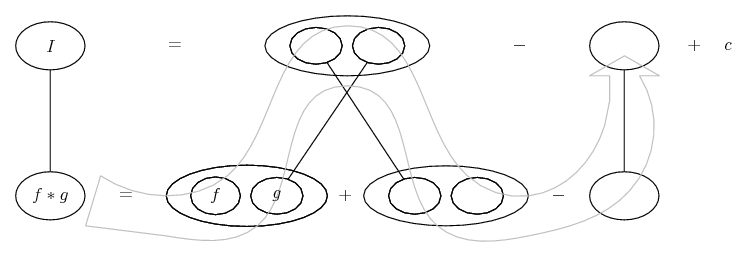... is lazy integration by parts, doing without u and v.... is the product rule, where straight lines differentiate downwards (integrate up) with respect to x.

Regarding one special way that Leibniz notation is suggestive in parts in particular, I'll locate an old thread if I can. (Edit: here) Anyway, though...

_________________________________________

Don't integrate - balloontegrate!

Balloon Calculus; standard integrals, derivatives and methods

Balloon Calculus Drawing with LaTeX and Asymptote!

5.Originally Posted by jameshumeHi TKH,

Thanks for your reply. Couple of typos there I hadn't spotted in my original post so no wonder your confusion at the first satement...
$\displaystyle \int \frac{dy}{dx} dx = y + C$

Starting with the product rule

$\displaystyle \frac{d}{dx}(uv) = v\frac{du}{dx} + u\frac{dv}{dx}$

So, again I made a mistake in what I had written, kinda, in that I should have written it as above, where y = uv.

So I would expect
$\displaystyle \int \frac{d}{dx}(uv) dx = uv$

I'm happy with that bit...

What I don't really get is
$\displaystyle \int u\frac{dv}{dx} dx$ becomes $\displaystyle \int u dv$?

I had a slight realisation when writing this and realised why it couldn't become $\displaystyle uv$, but equally I don't understand the apparent cancelling of the "d" terms. I could understand this if they were $\displaystyle \delta$s and therefore could be algebraically manipulated in this way.

I'm gonna go back to have a little think, but if you have any illuminating answers in the mean time I'd love to hear them.

THanks agin,
James
dv = (du/dx)dx is often taken as the definition of dv. naively, this means "a little bit of change in v" is v'(x) (the rate of change of v w.r.t. x) times "a little bit of change in x".

differentials aren't quite numbers, but some of the same rules apply.

the integral of v'(x) (or dv/dx) w.r.t. x (hence the "dx" which is for all intents and purposes a "dummy symbol" just to indicate which variable we're integrating a function of) is indeed v (+C).

however, the integral of (uv)' isn't uv, because we're not integrating over "d(uv)" but "dx". put another way, you can regard d(uv) as udv + vdu,

split the integral and you get 2, one where we're integrating u w.r.t. v, and another where we're integrating v w.r.t. u:

uv = ∫d(uv) = ∫(udv + vdu) = ∫udv + ∫vdu.

6.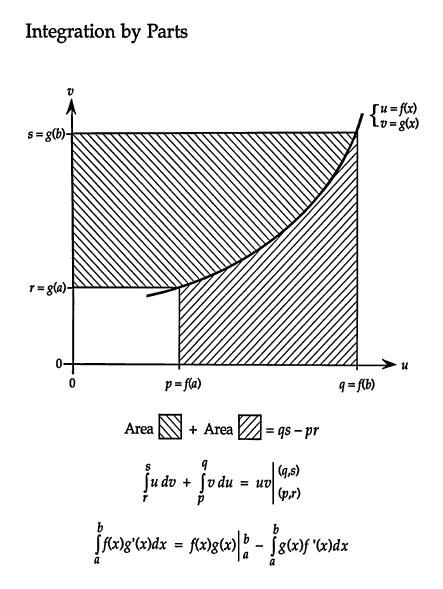7. ## Thanks

Hey Guys,

Wow, loads of replies so thank you to you all! I think a penny has dropped for me... Can someone verify the following for me so I can be confident I have properly understood

$\displaystyle \frac{d}{dx}(uv) = u\frac{dv}{dx} + v\frac{du}{dx}\\\\ \therefore uv = \int u\frac{dv}{dx} + \int v\frac{du}{dx}$

Then...
$\displaystyle \int u\frac{dv}{dx} = uv - \int v\frac{du}{dx}$
So the re-arrangement was fine. Then elsewhere I read the following...

For convenience can be memorised as...
$\displaystyle \int u.dv = uv - \int v.du$
In this form it is easier to remember, but the previous line gives its meaning in detail
Then having worked through some more examples I have understood better how the method works. My hang up was the line $\displaystyle \int u.dv = uv - \int v.du$. Am I correct in thinking that this is just a memory peg... I.e., written like that it doesn't mean integrate u w.r.t v, it's just a memory peg and the full meaning is integrate u*the differential coefficient of v with respect to x?? (Although given "Prove-it's graph I'm a little doubtful)

Thanks again for all your help guys... really appreciate it!

James

8.Originally Posted by jameshumeMy hang up was the line $\displaystyle \int u.dv = uv - \int v.du$. Am I correct in thinking that this is just a memory peg... I.e., written like that it doesn't mean integrate u w.r.t v, it's just a memory peg and the full meaning is integrate u*the differential coefficient of v with respect to x?? (Although given "Prove-it's graph I'm a little doubtful)
James
No, it can mean either way, i.e. either u w.r.t. v or else u times the derivative of v and all w.r.t. x. (Edit: all w.r.t. x as long as the dx is given as well, as Prove It has clarified below.) Prove It's graph helps to illuminate the relationship with a definite integral. And this one I made earlier also kind of fits his graph: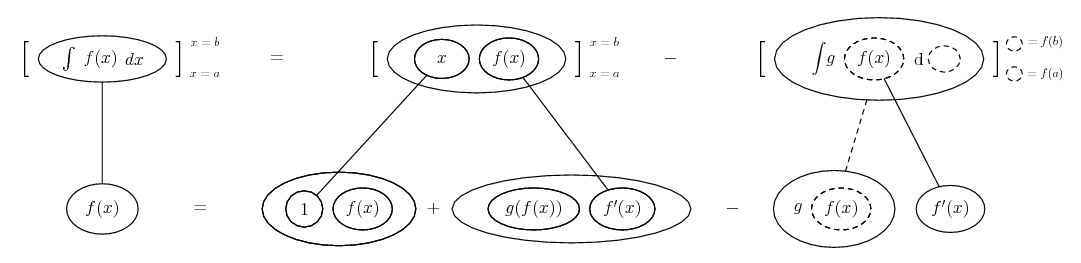See also the discussion I linked to above (the guy's question was very similar to yours).

9.Originally Posted by jameshume$\displaystyle \frac{d}{dx}(uv) = u\frac{dv}{dx} + v\frac{du}{dx}\\\\ \therefore uv = \int u\frac{dv}{dx} + \int v\frac{du}{dx}$
Should be $\displaystyle \displaystyle uv = \int{u\,\frac{dv}{dx}\,dx} + \int{v\,\frac{du}{dx}\,dx}$

Then...
$\displaystyle \int u\frac{dv}{dx} = uv - \int v\frac{du}{dx}$
Should be $\displaystyle \displaystyle \int{u\,\frac{dv}{dx}\,dx} = uv - \int{v\,\frac{du}{dx}\,dx}$.

I think you can see now why $\displaystyle \displaystyle \frac{dv}{dx}\,dx$ is shortened to $\displaystyle \displaystyle dv$ and why $\displaystyle \displaystyle \frac{du}{dx}\,dx$ is shortened to $\displaystyle \displaystyle du$.

10. the thing to remember is that u and v are not "independent variables" they both are functions of some other variable (we have been using "x").

so if u = e^x, for example, du is not "dx", but rather e^x dx. the key to using integration by parts, is to notice when "one part" is actually the derivative of something you already know.

11. Usually, yes...Originally Posted by Devenothe thing to remember is that u and v are not "independent variables" they both are functions of some other variable (we have been using "x").

so if u = e^x, for example, du is not "dx", but rather e^x dx. the key to using integration by parts, is to notice when "one part" is actually the derivative of something you already know.

... or, in other words...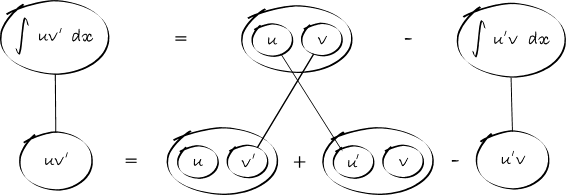(But you can use the balloons without u and v.)

On the other hand, one attraction of the fraction notation is that 'cancelling' is suggestive of how the chain rule does indeed allow us to read

$\displaystyle \int\ u\ dv = uv - \int v\ du$

as

$\displaystyle \int\ u\ \mathrm{wrt}\ v = uv - \int v\ \mathrm{wrt}\ u$

i.e.

$\displaystyle \int x\ \mathrm{wrt}\ y = xy - \int y\ \mathrm{wrt}\ x$

Just in case we didn't trust cancelling, here's another view of how the chain rule does it...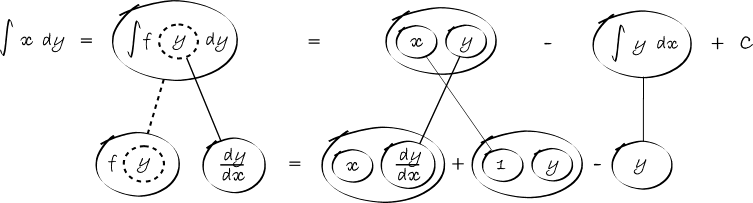,

,

,

,

,

,

,

,

# d(uv)/dx =udv/dx v(du/dx) show

Click on a term to search for related topics.

#### Search Tags

integration, integration proof parts, parts, proof